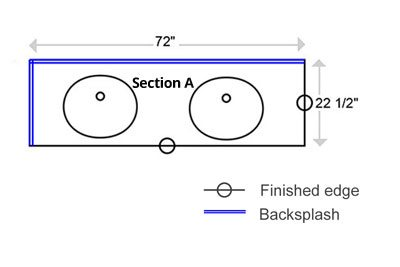• Mon - Fri ( 08:00 to 04:30 )
• Mon - Fri ( 08:00 to 04:30 )

## How to measure countertops

If you would like get accurate estimate for granite or quartz countertops, please use this page to give us the measurements of your countertop area, edge style, and the color of granite or quartz, and we will be happy to give you a free estimate.

## Below is a guide for drawing and measuring your countertop.

Make a layout of your project . Later you can upload it to one of our online estimate forms or you can just fax it or email it . Please provide us with as much information as you can, regarding your project. This includes: layout and measurements, granite color or/and quartz color , edge, personal information including the job address, contact information, and any other details that will help us determine the quote of the job.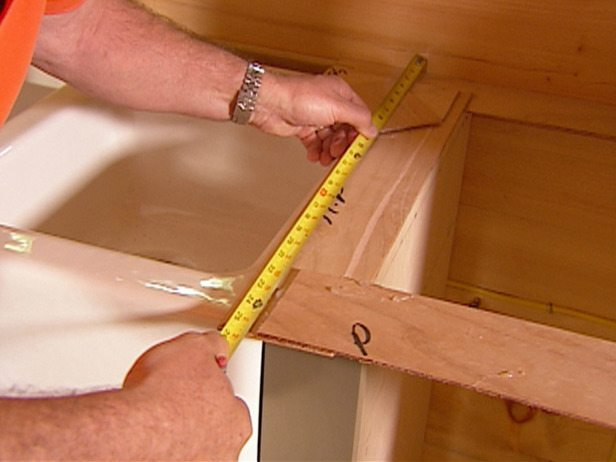• To start, be sure to measure from wall to wall. Don’t just measure your old countertops.
• Make a simple diagram of the counter layoutand transfer your measurements to it.
• Don’t worry if your measurements will be off – for quote inches doesn’t matter.
• Sink measurements doesn’t matter. Just draw where sink is more or less placed.
• If you have cooktop – you have to draw it too.
• Don’t forget to include your appliance locations!
• Use inches not feet (1 feet = 12 inches).
L - Shape Counter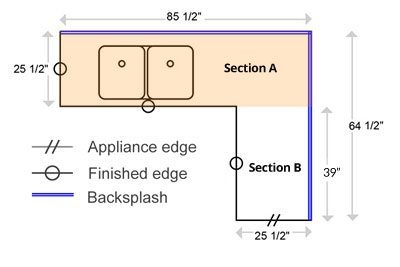• Example calculation for L – Shape Corner:
• Section A: 85.5 in x 25.5 in = 2180.25 sq in
• Section B: 39 in x 25.5 in = 994.5 sq in
• Backsplash: 85.5 in x 4 in = 342 sq in
• Backsplash: 64.5 in x 4 in = 258 sq in
• Backsplash: 64.5 in x 4 in = 258 sq in
• Total: 3774.75 sq in
• * Now divide by 144 to obtain square footage.
• Each sq foot has 144 sq in. (12 x 12).
• Total: 3774.75 sq in / 144 sq in = 26.21 sq ft
L - Shape Counter with Angle
• Example calculation for L – Shape Corner:
• Section A: 85.5 in x 25.5 in = 2180.25 sq
• Section B: 39 in x 25.5 in = 994.5 sq in
• Total: 3174.75 sq in
• * Now divide by 144 to obtain square footage.
• Each sq foot has 144 sq in. (12 x 12).
• Total: 3174.75 sq in / 144 sq in = 22.05 sq ftV - Shape Counter• Example calculation for V – Shape Corner:
• Section A: 60 in x 25.5 in = 1530 sq in
• Section B: 45 in x 25.5 in = 1147.50 sq in
• Total: 2677.50 sq in
• * Now divide by 144 to obtain square footage.
• Each sq foot has 144 sq in. (12 x 12).
• Total: 2677.50 sq in / 144 sq in = 18.59 sq ft
One Piece Counter
• Example calculation for – One Piece Counter:
• Section A: 31 in x 25.5 in = 790.5 sq in
• Section B: 31 in x 4 in = 124 sq in
• Total: 914.5 sq in
• * Now divide by 144 to obtain square footage.
• Each sq foot has 144 sq in. (12 x 12).
• Total: 914.5 sq in / 144 sq in = 6.35 sq ft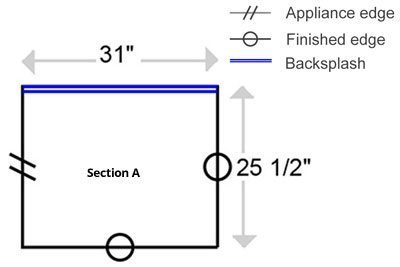Island Counter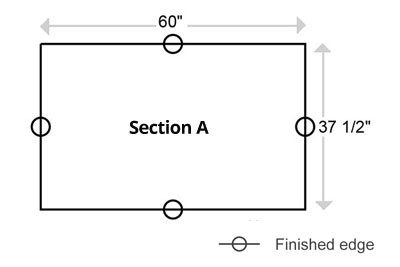• Example calculation for – Island Counter:
• Section A: 60 in x 37.5 in = 2250 sq in
• Total: 914.5 sq in
• * Now divide by 144 to obtain square footage.
• Each sq foot has 144 sq in. (12 x 12).
• Total: 2250 sq in / 144 sq in = 15.62 sq ft
One Piece Counter
• Example calculation for – Vanity Countertop:
• Section A: 72 in x 22.5 in = 1620 sq in
• Backsplash: 72 in x 4 in = 288 sq in
• Backsplash: 22.5 in x 4 in = 90 sq in
• Total: 1998 sq in
• * Now divide by 144 to obtain square footage.
• Each sq foot has 144 sq in. (12 x 12).
• Total: 1998 sq in / 144 sq in = 13.88 sq ft